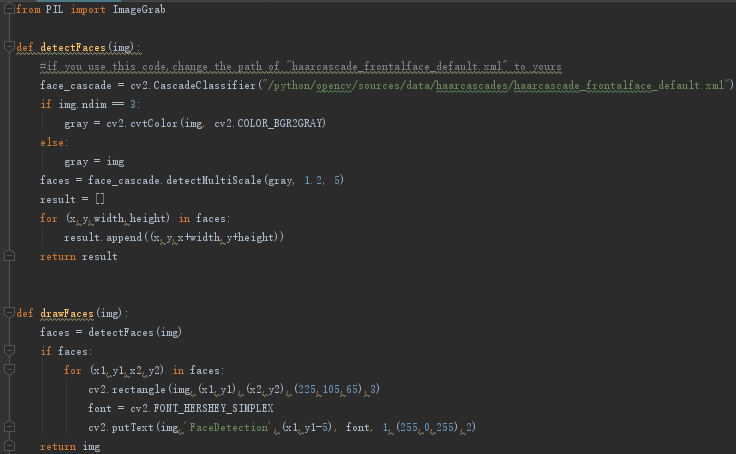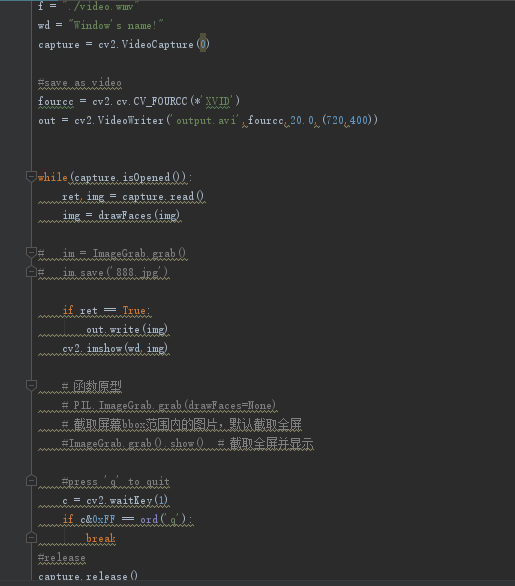python 人脸识别并截图保存 20Cpython + opencv人脸识别然后进行比对如何实现？

python + opencv人脸识别然后进行比对如何实现？ 现在能做的就是视屏流过来然后一帧一帧的判断进行人脸识别 能识别出来 但是如何把识别出来的人进行比对呢？ 例如视频中出现张三、李四人脸识别捕捉到了脸 但是怎么进行比对脸是谁？ 通过python实现 或是说python有什么库可以进行提取人脸特征然后保存

opencv人脸识别如何避免将xml加载到内存中

C++代码改Python实现PCA算法：从一组照片中获取特征脸（特征向量）？

PCA处理的基本步骤为： 1、获取m个样本，每个样本有n个特征。 2、每个样本作为一行，构成m*n的举证A。 3、将矩阵转置再乘以自己得到C=A(t)*A。 4、求出矩阵C的特征值及特征向量，特征向量即为特征脸。 以下是C++实现代码，求解决一份Python实现出的代码 ``` #include "stdafx.h" #include <string> #include <opencv2\opencv.hpp> using namespace std; using namespace cv; int _tmain(int argc, _TCHAR* argv[]) { //获取了mean_face int num_sample = 38; int norm_row = 64, norm_col = 56; int num; Mat imgs = loadImages(num); Mat mean_face = Mat(norm_row, norm_col, CV_8UC1); vector<int> mean_face_total; mean_face_total.resize(norm_row * norm_col); for (int i = 0; i < num; i++) { for (int j = 0; j < norm_row * norm_col; j++) { mean_face_total.at(j) += imgs.at<uchar>(i * norm_row * norm_col + j); //eigen_face_total[j] += imgs.at<uchar>(i * 192 * 168 + j); } } for (int j = 0; j < norm_row * norm_col; j++) { mean_face.at<uchar>(j) = (uchar)(mean_face_total.at(j) / num); } imwrite("C:/Users/dhj555/Desktop/YelaFaces/eigen_face/0001.jpg", mean_face); imshow("eigen_face", mean_face); //1、初始化数据 CvMat* pData = cvCreateMat(num_sample, norm_row * norm_col, CV_32FC1); CvMat* pMean = cvCreateMat(1, norm_row * norm_col, CV_32FC1); //每个数标志一个特征值 CvMat* pEigVals = cvCreateMat(1, min(num_sample, norm_row * norm_col), CV_32FC1); //每行表示一个特征向量 CvMat* pEigVecs = cvCreateMat(min(num_sample, norm_row * norm_col), norm_row * norm_col, CV_32FC1); for (int i = 0; i < num_sample; i++) { for (int j = 0; j < norm_row * norm_col; j++) cvmSet(pData, i, j, imgs.at<uchar>(i * norm_row * norm_col + j)); } //2、PCA处理 cvCalcPCA(pData, pMean, pEigVals, pEigVecs, CV_PCA_DATA_AS_ROW); //3、选出前P个特征向量(主成份),然后投影,结果保存在pResult中，pResult中包含了P个系数 //CvMat* pResult = cvCreateMat(num_sample, 20, CV_32FC1); //cvProjectPCA(pData, pMean, pEigVecs, pResult); //4、重构, 结果保存在pRecon中 //CvMat* pRecon = cvCreateMat(num_sample, norm_row*norm_col, CV_32FC1); //cvBackProjectPCA(pResult, pMean, pEigVecs, pRecon); //5、显示重构的图像 //Mat mRecon = Mat(pRecon); //4、显示与保存特征向量 for (int i = 0; i < min(num_sample, norm_row * norm_col); i++) { float min = LLONG_MAX, max = LLONG_MIN, span = 0.0; for (int index = 0; index < norm_row*norm_col; index++) { float d = cvmGet(pEigVecs, i, index); if (d>max) max = d; if (d < min) min = d; } span = max - min; Mat eigen_face = Mat(norm_row, norm_col, CV_8UC1); for (int index = 0; index < norm_row*norm_col; index++) { float d = cvmGet(pEigVecs, i, index); eigen_face.at<uchar>(index) = (d - min) / span * 255.0; } char buffer; sprintf_s(buffer, "C:/Users/dhj555/Desktop/YelaFaces/eigen_face/001/1-000%d.jpg", i); string imgPath(buffer); imshow(imgPath, eigen_face); printf("%d st:\t%f\n", i, cvmGet(pEigVals, 0, i)); imwrite(imgPath, eigen_face); } } ```

OPENCV图像归一化显示问题

opencv摄像头显示灰色

loonggg读完需要3分钟速读仅需 1 分钟大家好，我是你们的校长。我之前讲过，这年头，只要肯动脑，肯行动，程序员凭借自己的技术，赚钱的方式还是有很多种的。仅仅靠在公司出卖自己的劳动时...

MySQL数据库面试题（2020最新版）

HTTP与HTTPS的区别

《Oracle Java SE编程自学与面试指南》最佳学习路线图（2020最新版）

01、Java入门（Getting Started）;02、集成开发环境（IDE）;03、项目结构（Eclipse JavaProject）;04、类和对象（Classes and Objects）;05：词法结构（Lexical Structure）;06：数据类型和变量（Data Type and Variables）;07：运算符（Operators）;08：控制流程语句（Control Flow Statements）;

Java岗开发3年，公司临时抽查算法，离职后这几题我记一辈子

2020春招面试了10多家大厂，我把问烂了的数据库事务知识点总结了一下

2020年截止目前，我面试了阿里巴巴、腾讯、美团、拼多多、京东、快手等互联网大厂。我发现数据库事务在面试中出现的次数非常多。